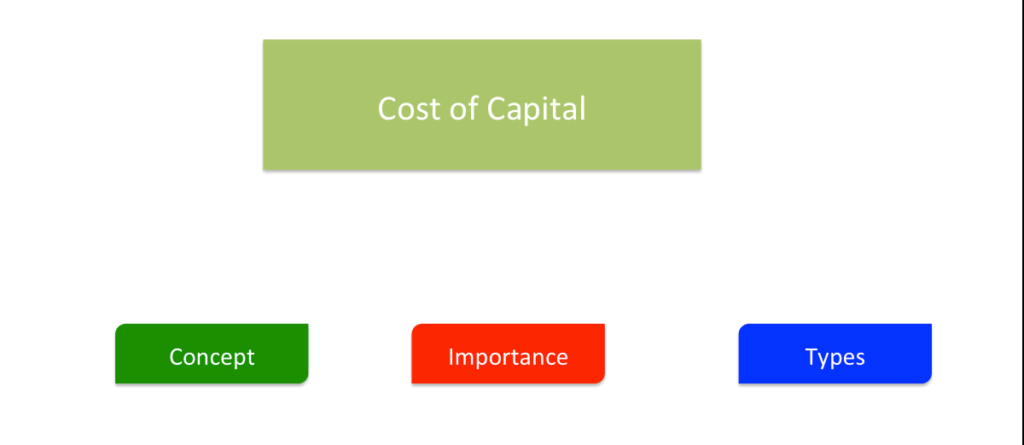# Cost of Capital: Concept, TypesThe cost of capital is the cost of a company’s funds (both debt and equity). In words of Solomon Erza “The cost of capital is the minimum required rate of earnings or the cut-off rate of expenditure”. From Investor’s point of view, it is the rate of return, that must be received by the firm on its investment projects, to attract investors for investing capital in the firm and to maintain its market value. In simple terms, it may be described  as the expected return appropriate for the expected level of risk. It is also termed as cut-off rate, the minimum rate of return, or hurdle rate.

### Importance

• Useful in determining company’s capital structure.
• Deciding future projects/investments – For an investment to be worthwhile, the expected return on capital has to be higher than the cost ofcapital.
• Helps to evaluate a company’s investment program and its competitive position.
• Helpful in capital budgeting decisions regarding the sources of finance used by the company.

#### Cost of Capital and Capital Structure

Cost ofcapital is an important factor in determining the company’s capital structure. Companies look for the optimal mix of financing (Debt+Equity) that provides adequate funding and that minimizes the cost ofcapital.

### Affecting Factors

• Capital Structure
• Dividend Policy
• Financial and Investment Decisions
• Interest Rates

### Types

#### Implicit & Explicit Capital Costs

• Implicit cost also known as the opportunity cost is the of the opportunity foregone in order to take up a particular project.  It is the rate of return associated with the best investment opportunity for the firm and its shareholders that will be forgone if the project presently under consideration by the firm were accepted.
• Explicit cost of any source may be defined as the discount rate that equates the present value of the funds received by a firm with the present value of expected cash outflows.

#### Historical & Future Capital Costs:

• Historical costs are those costs which have already been incurred in order to finance a particular project.
• Future Costs are the expected costs of funds for financing a particular project.

### Weighted average cost of capital (WACC)

WACC is the combined cost of each component of funds raised by the firm. The weights are the proportion of the value of each component of capital in the total capital.

### Marginal Capital Costs

Marginal capital cost is defined as the cost of obtaining one additional unit (1 rupee in India) of new capital.

### Specific Capital Cost:

The cost of each component of capital is known as specific Capital Costs. Companies raise capital from different sources such as equity, debentures, loan etc. It is the cost of equity capital, cost of debentures, etc., individually.

#### Cost of Debt

• It is the interest rate that a company pays on its existing debt.
• However, since interest expense is tax-deductible, the debt is calculated on an after-tax basis as follows:
Cost of Debt = Interest Expense * ( 1- Tax Rate)/ Total Debt

#### Cost of Equity:

• It is the expected rate of return for the company’s shareholders.

#### Cost of Retained Earnings:

• Retained earnings refers to the portion of net income which is retained by the corporation rather than distributed to its owners as dividends.

Reference:

error: Content is protected !!